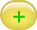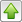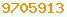# precedence : Java Glossary

precedence

Java inherits a very complicated operator precedence scheme from C. Operators with lower precedence numbers are evaluated first. The advantage of precedence is it means you need fewer parentheses. The disadvantage is you must memorise this table perfectly to understand what code will do. Java does not consider ( ) { } [ ] ;, . as operators. They are classed as separators.

Once
`x = a | b & c;`
means:
`x = ( a | b ) & c;`
or
`x = a | ( b & c );`
Operators near the top of the table are evaluated first. The lower the precedence number, the earlier the operations are done. Equal precedence operations usually are evaluated left to right, except for ones with right association, which are evaluated right to left. Another way of putting this is right-associated operators like ? have the deepest implied nesting on the right. What a hodge podge!

## Tips

• If you are not sure about precedence or left-right association, add sufficient parentheses to make your meaning clear. If you are confused, likely others reading your code will be too.
• Use Intellij Idea IDE (Integrated Development Environment) to remove unnecessary parentheses.
• The most common error I make is an expression of this form:
```// Beware of code like this:
b =  a & 0xfff + 1;

// Java treats this as:
b =  a & ( 0xfff + 1 );

// not
b = ( a & 0xfff ) + 1;
// which you likely intended```
Java Operator Precedence
Operators near the top are evaluated first.
Precedence Operator Association Notes
0 new Right new, according to the JLS is not officially an operator. It is not usually considered to have precedence since it can occur in only one context, immediately followed by a class name. IIRC (If I Recall Correctly) it was considered to be an operator in the Java 1.0 days.
1 (prefix) ++
(prefix) --
(unary) +
(unary) -
(unary) ~
(unary) !
(cast)
Right (prefix) ++ prefix means preincrement (add 1).-- prefix means predecrement (subtract 1).Preincrement increments before sampling the value to use in evaluating the rest of the expression. ! is logical not for booleans. Nearly always, you have to put the expression after ! in parentheses. You might as well make a habit of always doing it.(cast) and parenthesis are not officially operators, according the JLS .~ tilde is bitwise not for ints.(cast) has a multitude of purposes, lumped together under the term casting1 (postfix) ++
(postfix) --
Right (postfix) ++ postfix means postincrement (add 1).-- postfix means postdecrement (subtract 1 ).Postincrement increments immediately after sampling the value to use in evaluating the rest of the expression.
Be very careful about using a pre/post inc/decremented variable elsewhere in an expression. You may be in for a surprise. for example
```// Be very careful reusing a pre or post increment variable in an expression.
int x = 2;
int y = x++ * ++x;  // result is y = 8;

// it works as if you had coded:
int x = 2;
int a = x++;   // a=2; x=3;
int b = ++x;   // b=4; x=4
int y = a * b; // y = 8```
2 *
/
%
Left (infix) * is integer multiplication for ints and floating pointmultiplication for doubles.% is the remainder operator, informally and incorrectly called the modulus operator./ is integer division for ints and floating point division for doubles.3 +
-
Left (infix) + is ordinary addition, integer or floating point..
- is ordinary subtraction, integer or floating point..
a - b - c means (a - b) - c not a - ( b - c ), additive operations are performed left to right. + also means concatenation.
4 <<
>>
>>>
Left (infix) There is no <<< operator because it would be identical to <<. You have to keep your wits about you when doing unsigned shifts to remember all right shifts must be done with >>>. In Java version 1.5 or later the following methods are built-in letting you avoid much low-level bit fiddling: e.g. Integer.highestOneBit, lowestOneBit, numberOfLeadingZeros, numberOfTrailingZeros, bitCount, rotateLeft, rotateRight, reverse, signum and reverseBytes..
5 <
>
<=
>=
instanceof
Left (infix) oddly instanceof is considered an operator, even though it cannot operate on expressions.6 ==
!=
Left (infix) == is for comparison. != means not equal.= is for assignment. Pascal’s <> not equal will not work. == and != work on booleans too, often saving a forest of if/elses.
7 & Left (infix) Bitwise AND masking mostly for for ints.8 ^ Left (infix)

XOR (exclusive OR) for ints. It is the difference operator. It is true if the boolean operands are different, e. g.

```false ^ false == false
false ^ true == true
true ^ false == true
true ^ true == false```

It is useful in cryptography because of this magic property of encryption and decryption with a random scrambler number.

```long encrypted = message ^ scrambler;
long decryped = encrypted ^ scrambler;```

If you XOR twice with the scrambler, you get right back where you started. For booleans it is clearer to use a != b instead of a ^ b and a == b instead of !( a ^ b)9 | Left (infix) bitwise OR mostly for ints.
```int e = ( a & b << 2 ) | ( c & ( d >>> 1 ) );
// if you trust precedence can be written more tersely:
int e = a & b << 2 | c & d >>> 1;```10 && Left (infix) short circuit logical AND for booleans.11 || Left (infix) short circuit logical OR for booleans. So when you say:
`if ( ( ( lowest <= a ) && ( a <= biggest ) ) || notNeeded )`

all those parentheses are nugatory (computer jargon for not necessary but won’t hurt). You could just as easily have written it, trusting precedence, as:

`if ( lowest <= a && a <= biggest || notNeeded )`12 ? : Right (ternary)13 =
*=
/=
+=
-=
<<=
>>=
>>>=
&=
^=
|=
Right (infix) a += b means a = a + b; a *= b means a = a * b; etc. These make proofreading easier by eliminating typing a variable name twice.The most important extra rule is that parameters to a method are evaluated left to right.

## Learning MoreThis page is postedon the web at: http://mindprod.com/jgloss/precedence.html Optional Replicator mirror of mindprod.com on local hard disk J: J:\mindprod\jgloss\precedence.htmlPlease read the feedback from other visitors, or send your own feedback about the site.Contact Roedy. Please feel free to link to this page without explicit permission. Canadian Mind Products IP:[65.110.21.43] Your face IP:[35.172.165.64] Feedback You are visitor number